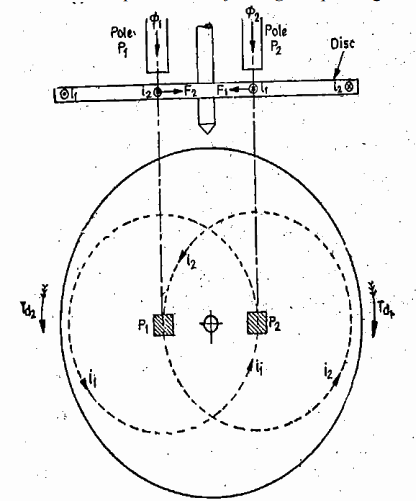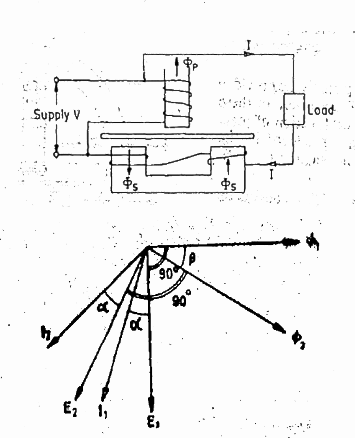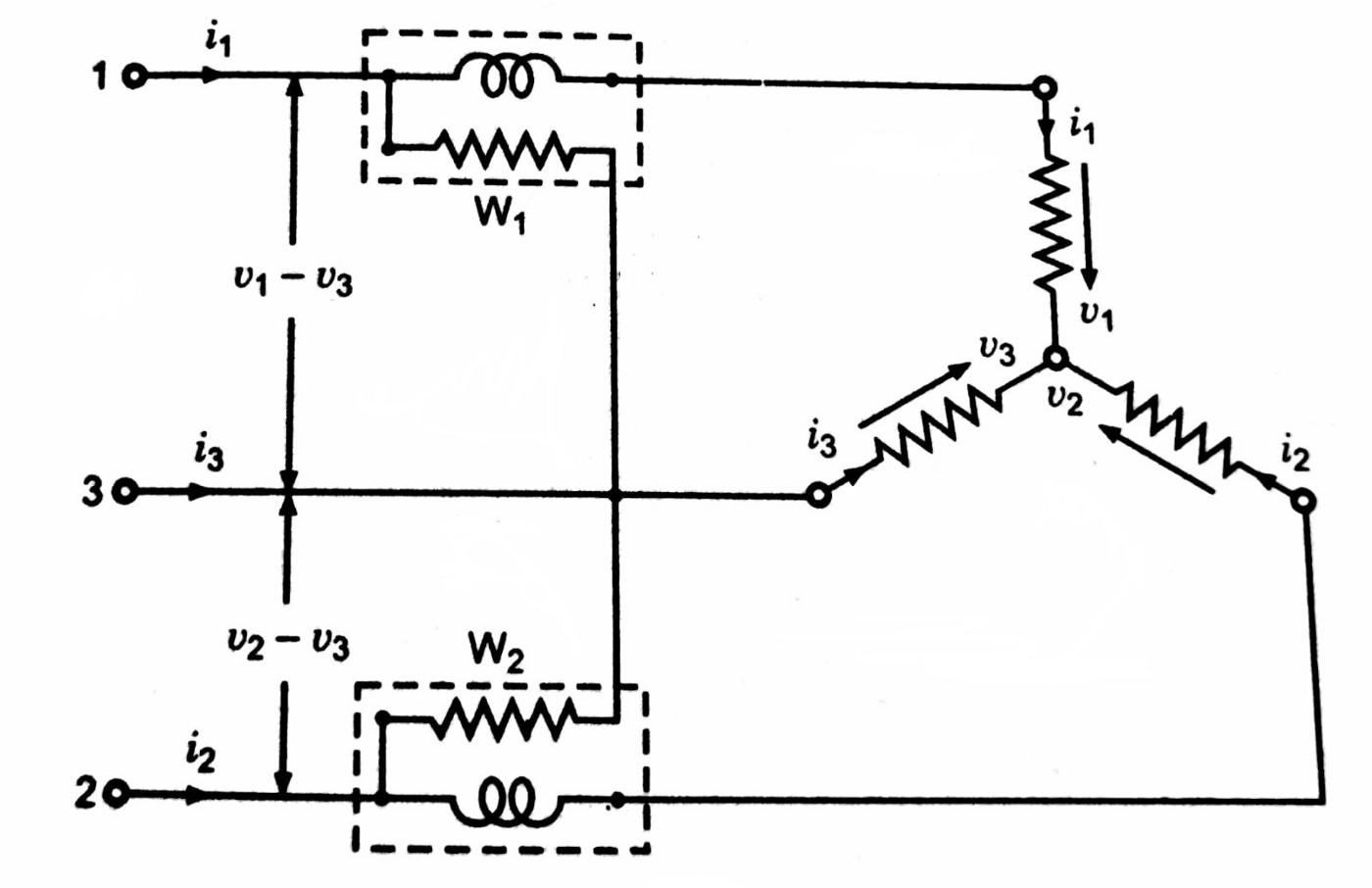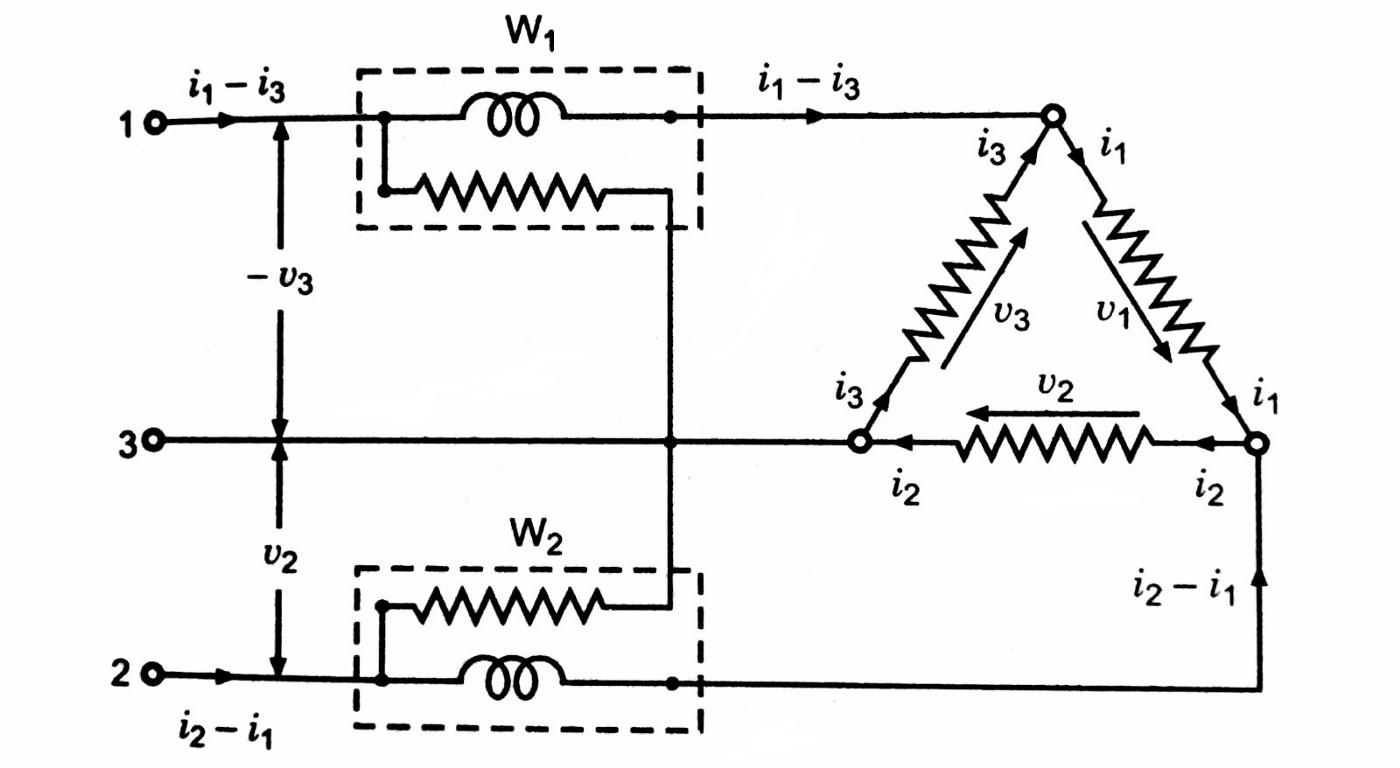## Operating Principle of Single Phase Energy Meter

1. In the construction part, we discussed the different parts of the single phase energy meter. We also saw that we have two currents flowing in the system, one of the current is proportional to the Voltage of the supply and flowing in the pressure coil, the second one is flowing in the current coil and is proportional to the supply current (Refer to the figure under the heading “Single Phase Induction Type Watt-Hour Meter”). Since both the currents are alternating in nature so the flux produced by both the currents is also alternating in nature. When these alternating flux pass through the Aluminium disc, which is placed between two coils, an emf is generated in the disc, this emf causes the eddy currents to flow in the disc. These eddy currents flow in a small circular path and produces torque of their own. Thus we have two eddy currents causing two torques. The resultant of these two torques is the Deflecting Torque. Fig.  below depicts this operation. The two type of fluxes Φ1 and Φ2 are the same as Φp and Φs as shown in the figure. They produce i1 and i2 eddy currents which in turn produce Td1 and Td2 deflecting torques. These torques produce F1 and F2 force on the disc which in turn rotate the disc. Since the two torques are proportional to the two alternating fluxes, which are proportional to the voltage and current of the supply respectively, we have the rotation of the disc proportional to the power. The registering system measures the number of rotation of the disc in a particular amount of time and converts the Power into Energy by multiplying with time. The scale is calibrated to show the energy consumed.1. Three Phase Energy Measurement Just like 3- Φ power measurement, here also we can use 3 energy meters for 3- Φ 4-wire system and 2 energy meters for 3- Φ 3 wire system. The assembly with 2 energy meters is most common because of the ease of operation and also because of the wide use of the 3 wire system. Figure below shows the two energy meter method for the measurement of 3- Φ energy.The meter shown above is provided with 2 discs, one for each element. It is essential that the driving torque of the two elements be exactly equal for equal amounts of power passing through each phase. Thus in addition to normal compensating devices attached to each element, an adjustable magnetic shunt is provided on one or both the elements to balance the torques of the two. The pressure coils are connected in parallel and the current coils in series in such a manner that the torques produced by the two elements oppose each other. The magnetic shunt is adjusted to a position where the two torques are exactly equal and opposite and therefore there is no rotation of disc. This way the two elements are rendered exactly similar.

2. Errors in the Energy Meter:
The errors in an energy meter are predominantly due the driving system. Some of the important errors due to driving system are:
Incorrect magnitude of fluxes: This may be due to abnormal values of current or voltage. The shunt magnet flux may be in error due to changes in resistance of coil or due to abnormal frequencies.
Incorrect phase angles: There may not be proper relationship between the various phasors, this may be due to improper lag adjustment, abnormal frequencies, change in resistance with temperature, etc.
Lack of Symmetry in magnetic circuit: In case the magnetic circuit is not symmetrical, a driving torque is produced which makes the meter creep.
The errors due to the braking system are:

1. Changes in strength of brake magnet

2. Changes in disc resistance

3. Self-braking effects of series magnet flux

4. Abnormal friction of moving parts

The adjustments required to rectify the errors which are described above are:
1. Preliminary light load adjustment: The disc is placed in such a manner that the holes are not underneath the electromagnets. Rated voltage is applied to the potential coil with no current through the current coil. The lightload device is adjusted until the disc just fails to start.
2. Full load Unity Factor Adjustment: The pressure coil is connected across the supply voltage and rated full load current at unity power factor is passed through the current coils. The position of the brake magnet is adjusted to vary the braking torque so that the meter revolves at the required speed within required limits or error.
3. Lag Adjustment (Low power factor adjustment): The pressure coil is connected across rated supply voltage and rated full load current is passed through the current coil at 0.5 p.f. lagging. The lag device is adjusted, till the meter runs at correct speed.
4. Light Load Adjustment: Rated supply voltage is applied across the pressure coil and a very low current (about 5 percent of full load) is passed through the meter at unity power factor. The Light load adjustment is done so that the meter runs at the correct speed.
5. Creep Adjustment: As a final check on light load adjustment, the pressure coil is excited by 110 percent of rated voltage with zero load current. If the light load adjustment is correct, the meter should not creep under these conditions.

## Two wattmeter method is generally used for measurement of power in 3-phase,3 wire load circuits. The current coils of two wattmeters are inserted in any two lines and pressure coil is connected from its own current coil to the line without a current coil.Let V1,V2,V3 and I1,I2,I3 be the voltages and currents of the three loads connected across three different phases at particular instant. Thus the power at the instant under consideration is equal to the sum of their products regardless of power factor.

Measurement of Power by Two Wattmeter Method in Star Connection systemTwo-wattmeter method of measuring 3-phase 3-wire power in Star connected system

According to the Kirchhoff's first law,the algebraic sum of three instantaneous current is Zero as all three phases meet at a star point is the instantaneous power measured by wattmeter W1.since i2 is the instantaneous current flowing through the current coil and (v2-v3) is the instantaneous potential difference across pressure coil of wattmeter W2, is the instantaneous power measured by wattmeter W2.

P=W1+W2Measurement of Power by Two Wattmeter Method in Delta Connection system
Two-wattmeter method of measuring 3-phase 3-wire power in Delta- connected system

In delta connected system the three phases form a closed loop,according to Krichhoff`s second law ,

v1+v2+v3=0

Instantaneous Power(P)=V1I1+V2I2+V3I3

since -v3 is the instantaneous potential difference across pressure coil and (i1-i3) is the instantaneous current flowing through current coil of wattmeter W1,

since v2 is the instantaneous potential difference across pressure coil and (i2-i1) is the instantaneous current flowing through current coil of wattmeter W2,

W2=V2(I2-I1)